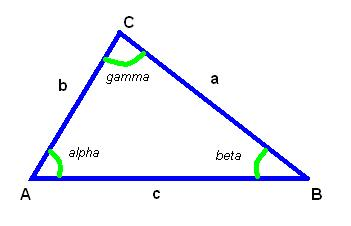# Sine Law Calculator

This online calculator determines the lengths of triangle sides given one side and two angles using the law of sines.The law of sines, also known as the sine rule, states that the ratio of the length of a side of a triangle to the sine of the opposite angle is constant for all three sides and angles.

The calculator takes as input the length of one side of the triangle, and the values of two angles adjusted to this side. It then uses the law of sines to calculate the lengths of the other two sides of the triangle.#### Lengths of triangle sides given one side and two angles

°
°
Digits after the decimal point: 2
Side A

Side B

URL copied to clipboard

#### Similar calculators

PLANETCALC, Sine Law Calculator